# Assignments

This series of exercises is about exponential functions and their graphs. We discuss the various aspects: what is an exponential function, how does the graph looks like and how can you make a sketch of the graph without using a graphing calculator. We also make a start with solving exponential equations if you want to calculate the intersection of two exponential functions.

Select a question by clicking on it.

1. Draw the graph of the function: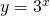What is the formula of the asymptote?

Solution

2. Draw the graph of the function: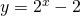What is the formula of the asymptote?

Solution

3. Draw the graph of the function: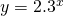Calculate the intersection point with the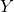-axis and the equation of the asymptote.

Solution

4. Draw the graph of the function: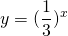What is the formula of the asymptote?

Solution

5. Draw the graph of the function: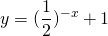What is the formula of the asymptote?

Solution

6. Draw the graph of the function: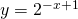What is the formula of the asymptote?

Solution

7. Draw the graph of the function: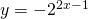What is the formula of the asymptote?

Solution

8. Draw the graph of the function: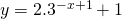What is the formula of the asymptote?

Solution

9. Draw the graphs of the functions: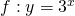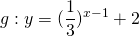Calculate the intersection point of both graphs.

Solution

10. For which value(s) of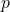do the graphs of the functions: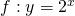have only one point in common.

Solution

0